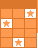Challenge Board: Score points by answering questions correctly.# Division Facts III: Mixed Review and Word Problems

See if you know the quotient (answer) for these basic division problems. Since I do not have the traditional division symbols on my computer, "divided by" is expressed as "/" (For example, "18 / 6" means "18 divided by 6"). For the word problems, write the equation that helped you determine your answer, leaving a space between each number and symbol (For example, "18 / 6 = 3").This activity was created by a Quia Web subscriber. Learn more about QuiaCreate your own activities